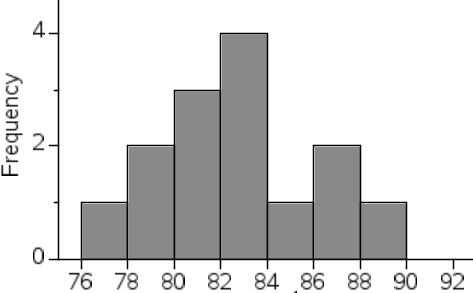# 2106 Univariate Data - Creating Intervals

Approved & Edited by ProProfs Editorial Team
The editorial team at ProProfs Quizzes consists of a select group of subject experts, trivia writers, and quiz masters who have authored over 10,000 quizzes taken by more than 100 million users. This team includes our in-house seasoned quiz moderators and subject matter experts. Our editorial experts, spread across the world, are rigorously trained using our comprehensive guidelines to ensure that you receive the highest quality quizzes.
| By Anthony Nunan
A
Anthony Nunan
Community Contributor
Quizzes Created: 159 | Total Attempts: 46,613
Questions: 18 | Attempts: 693SettingsHow do you create an interval? How do you know how wide it should be, and how many intervals to create?

• 1.

### What is the ideal number of intervals for a set of data?

• A.

Less than 5

• B.

More than 20

• C.

12

• D.

Between 5 and 15

• E.

Less than 7

• F.

I don't know

D. Between 5 and 15
Explanation
The ideal number of intervals for a set of data is between 5 and 15. This range allows for a sufficient level of detail in the data without overwhelming the reader with too many intervals. Having fewer than 5 intervals may result in oversimplification and loss of important information, while having more than 15 intervals may make it difficult to interpret the data accurately. Therefore, a range of 5 to 15 intervals strikes a balance between providing enough detail and maintaining clarity.

Rate this question:

• 2.

### The number of intervals we choose for a data set is 'arbitrary'. What does this mean?

• A.

Exact

• B.

Small

• C.

Randomly chosen

• D.

Chosen by a formula

• E.

Designed to confuse

• F.

I don't know

C. Randomly chosen
Explanation
This means that the number of intervals selected for a data set is not predetermined or based on a specific formula or criteria. Instead, it is chosen without any specific pattern or rule, making it a random selection.

Rate this question:

• 3.

### The interval width we choose is designed to :

• A.

Show all the data

• B.

Show a pattern in the data

• C.

Display the data laterally

• D.

Show the range more easily

• E.

• F.

I don't know

B. Show a pattern in the data
Explanation
The interval width we choose is designed to show a pattern in the data. By selecting an appropriate interval width, we can group the data into meaningful categories or ranges, which allows us to identify any patterns or trends that may exist in the data. This helps in analyzing and interpreting the data more effectively, as it highlights any regularities or relationships that might be present.

Rate this question:

• 4.

### I have data from 200 people regarding their resting heart rates - from 47 beats per minute to 108 beats per minute. If I decide to make the intervals 10 wide, how many intervals would I have?

7
Explanation
108 - 47 = 61 ... the range is 61. So, divide by 10, that gives you 6.1 - so it is likely to be 7, possibly 8. Test it.
Starting at 40-49.9, 50-, 60- 70-, 80-, 90-, 100-109.9

Rate this question:

• 5.

### I have data from 200 people regarding their resting heart rates - from 67 beats per minute to 108 beats per minute. If I decide to make the intervals 10 wide, how many intervals would I have?

5
Explanation
108 - 67 = 41 ... the range is 41. So, divide by 10, that gives you 4.1 - so it is likely to be 5, possibly 6. Test it.
Starting at 60-60.9, 70-, 80-, 90-, 100-109.9

Rate this question:

• 6.

### What is the width of the interval class shown in the histogram above

2
Explanation
The width of the interval class shown in the histogram is 2. This means that each interval in the histogram has a width of 2 units.

Rate this question:

• 7.

### The histogram below displays a set of data in interval classes. The first class goes from 76-77.9, the second from 78-79.9 etc.What is the modal class interval?

82-83.9
Explanation
The modal class interval is the interval that has the highest frequency or the tallest bar in the histogram. In this case, the given histogram does not provide any information about the frequencies of the intervals. Therefore, it is not possible to determine the modal class interval based on the given information.

Rate this question:

• 8.

### What is the width of the interval class shown in the histogram above?

1
Explanation
The width of the interval class shown in the histogram above is 1. This means that each interval in the histogram has a width of 1 unit.

Rate this question:

• 9.

5
• 10.

### How many interval classes are in the histogram above?

7
Explanation
The histogram above shows the distribution of data across different intervals. Each interval represents a range of values. By counting the number of intervals shown in the histogram, we can determine the number of interval classes. In this case, there are 7 intervals shown, indicating that there are 7 interval classes in the histogram.

Rate this question:

• 11.